• Implement all pieces together to make overall process for neural networks learning algorithm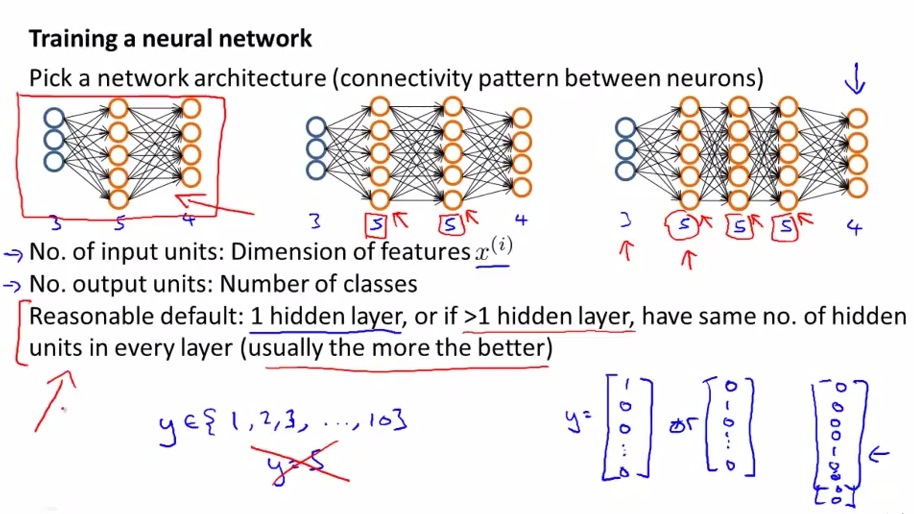• find option pattern for neural network
• Multiclass classification is the number of classes in output units
• 1 hidden layer is the most common
• if chose more than 1 hidden layer, every hidden layer should be same number of hidden units
• more hidden layer could be computationally expensive, but sometimes a good things
• How to choose number of hidden layer or number of hidden units will be discussed later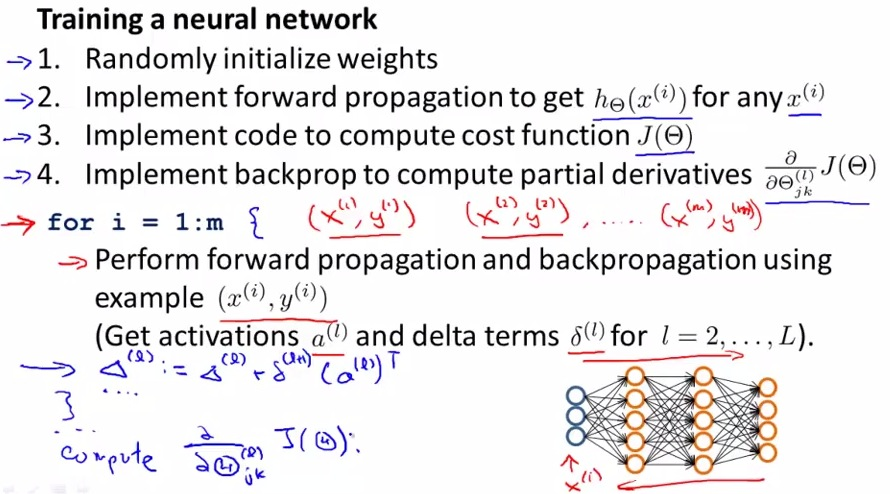• These are the steps to implement a neural network
• First we need the matrix weight, because it is affected by the backprop and backprop is not allowed for the first example,
• then we random initialized the matrix weight because each must not be the same. Use epsilon as a boundary random.
• Then we using forward propagation until we get the final cost function
• use forward and backprop for every example; forward  backprop first example, go to second example, use forward and backprop and so on until we reached the final example
• Not recommend using without for loops if we first try to backprop
• So for every iteration examples, go through layer, forward and backprop, compute the delta, doing so until reach the final iteration examples
• Then write some code, then finally compute partial derrivative of cost function, using regularization that we defined earlier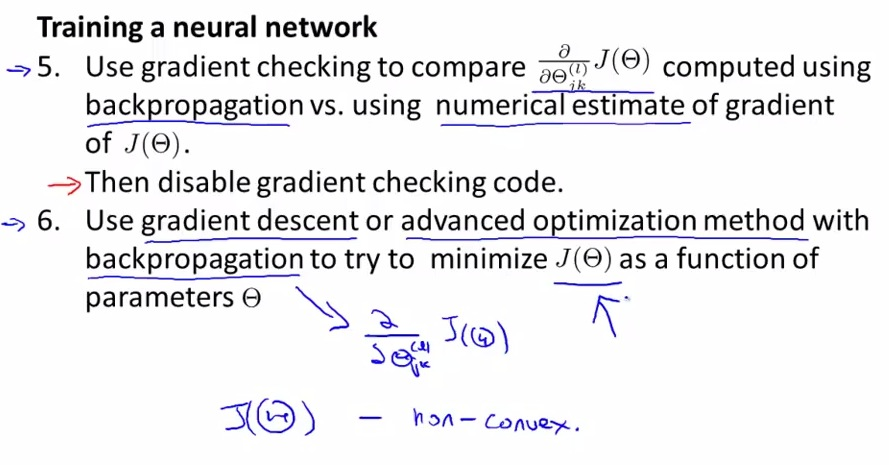• gradient checking to make sure that the code we implemented is correct
• use advanced optimization or other techniques to try to minimize the cost function of j(theta)
• Actually the big j(theta) is actually non-convex, so sometimes it find itself in local optima, but often it doesn't find much problem, as it tends to find a global optima
• If it does still feel magical for gradient descent in nerural networks, the graph below will help to visualize better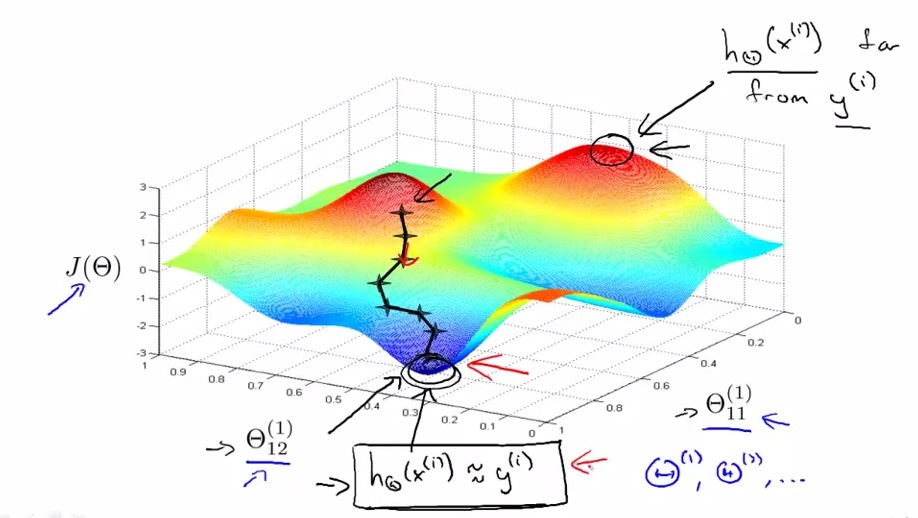• here are just examples with three axis Cost Function, first parameters, and second parameters respectively
• Of course in reality there's tend to be a lot of parameters
• The cost function will be pretty low(valley) , resulting with hyphotesis is approach(similar to) y, where hill result hyphotesis far from y
• The backprop at init will determining the direction to go to the global optima, and the gradient descent will continue the rest of the step to find itself in global optima
• So what the backprop or gradient descent or advance techniques are doing, is to find itself a hyphothesis output value that matche with output at the training set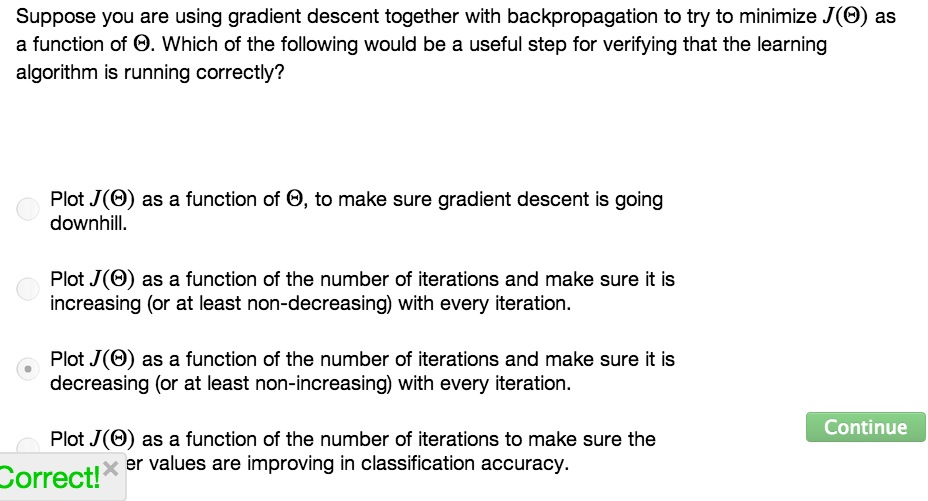• In conclusion, these hopefully helps better to visualize as well as take a bigger picture on how to implement steps in neural networks learning algorithm as a whole
• Much harder than linear regression and logistic regression
• Nevertheless the most powerful learning algorithm for non-linear function to date